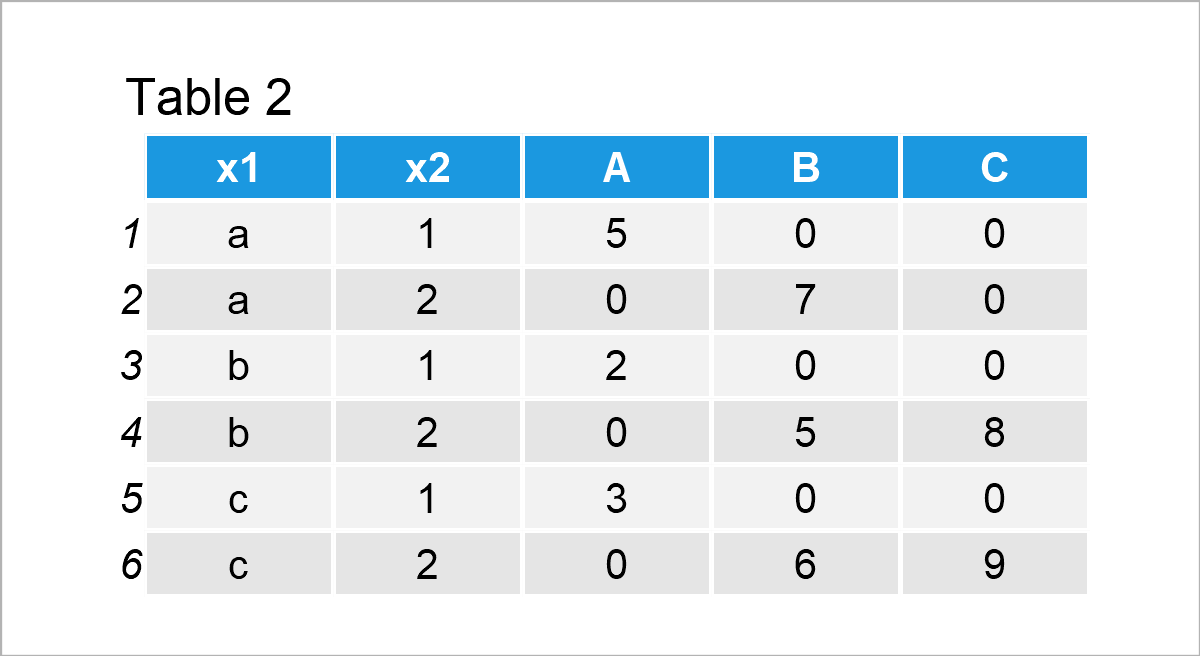# How to Create a Pivot Table in R (Example)

In this tutorial you’ll learn how to make a pivot table in R.

You’re here for the answer, so let’s get straight to the R code…

## Creating Example Data

First and foremost, we’ll need to create some example data:

```data <- data.frame(x1 = letters[1:3],    # Create example data frame
x2 = c(rep(1:2, each = 4), 2),
x3 = 1:9,
x4 = c(rep("A", 4), rep("B", 3), rep("C", 2)))
data                                     # Print example data frame```As you can see based on Table 1, our example data is a data frame made up of nine rows and four columns. The variables x1 and x4 have the character data class, the variable x2 has the numeric data type, and the variable x3 has the integer class.

Let’s create a pivot table as in Microsoft Excel using these data!

## Example: Make Pivot Table Using dcast() Function of reshape2 Package

In this example, I’ll illustrate how to create a pivot table in the R programming language.

First, we have to install and load the reshape2 package:

```install.packages("reshape2")             # Install & load reshape2
library("reshape2")```

Next, we can apply the dcast function to create a pivot table:

```data_pivot <- dcast(data,                # Create pivot table
x1 + x2 ~ x4,
value.var = "x3",
fun.aggregate = sum)
data_pivot                               # Print pivot table```Table 2 shows the output of the previous R code, i.e. a pivot table where we have used multiple columns (x1, x2, and x4) to define the groups in our table. The variable x3 provided the values for the pivot table.

## Video & Further Resources

Do you need further explanations on the R programming syntax of this article? Then I can recommend having a look at the following video that I have published on my YouTube channel. I’m showing the R programming code of the present tutorial in the video.

Furthermore, you may want to read the related articles on my homepage:

This tutorial has demonstrated how to create a pivot table in the R programming language.

In this tutorial, we have used the reshape2 package to create a pivot table. In case you want to create pivot tables with packages such as dplyr and tidyr, you may have a look at the pivot_longer and pivot_wider functions.

Please let me know in the comments, in case you have any further questions.

Subscribe to the Statistics Globe Newsletter